# Pontryagin character

(diff) ← Older revision | Latest revision (diff) | Newer revision → (diff)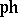The characteristic class defined by the equality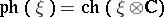, where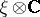is the complexification of the fibre bundleand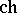is the Chern character. The Pontryagin character as an element of the ringis determined by the even series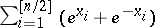and has the following properties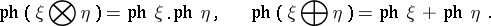The index class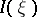is defined to be equal to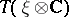, where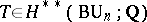is the Todd class. The index class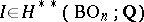is expressed in Wu generators (see Characteristic class) by the formulaThe following theorem on the relationship between the Pontryagin class and the-class holds (cf. Pontryagin class). Letbe a real vector bundle over the basewith a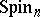-structure,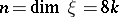. For such bundles there is the Thom isomorphism in real-theory:Let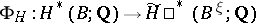be the Thom isomorphism, which is uniquely determined by the orientation of the bundle. Then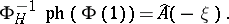This formula is an exact analogue of the corresponding statement on the relationship between the Chern character and the Todd class.

Ifis a complex vector bundle, then. Here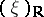is the real part of the bundle, andis the Todd class.

For references see Pontryagin class.

How to Cite This Entry:
Pontryagin character. Encyclopedia of Mathematics. URL: http://encyclopediaofmath.org/index.php?title=Pontryagin_character&oldid=16666
This article was adapted from an original article by A.F. Kharshiladze (originator), which appeared in Encyclopedia of Mathematics - ISBN 1402006098. See original article